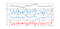# Fusing the RSI with the Stochastic Oscillator. Does It Improve the Trading Results?

## Coding an RSI-Stochastic Indicator for Systematic Trading and Back-testing the Results.

Trading is a numbers game and the more confirming elements we have, the higher our conviction is to take the position. In this article, we will discuss two famous indicators, namely, the RSI and the Stochastic oscillator, and see whether we can fuse them together to form a new one. If you feel like creating your own technical indicator from scratch, check out this article I have written recently here on Medium:

Now, back to our topic. First, we will quickly introduce the two indicators and then present the framework that we will follow to create the new RSI-Stochastic indicator which will be later used to generate trading signals.

The Stochastic oscillator seeks to find oversold and overbought zones by incorporating the highs and lows as well in its formula. It uses the below formula to calculate its values:

We will create the below function that calculates the Stochastic on an OHLC data:

`def stochastic(Data, lookback, onwhat, where):            for i in range(len(Data)):                try:                Data[i, where] = (Data[i, onwhat] - min(Data[i -  lookback:i + 1, 2])) / (max(Data[i - lookback:i + 1, 1]) - min(Data[i - lookback:i + 1, 2]))        except ValueError:            pass        Data[:, where] = Data[:, where] * 100return Data`

The Relative Strength Indicator -RSI- has been created by J. Welles Wilder in 1978 as a momentum indicator with an optimal look-back period of 14 bars. It is bounded between 0 and 100 with 30 and 70 as the agreed-upon oversold and overbought zones respectively. The RSI can be used through 4 known techniques:

• Oversold and overbought zones as indicators of possible short-term corrections or reversals.
• Divergence from prices as an indication of trend exhaustion.
• Drawing normal supports/resistances on the indicator just as we would do with prices.
• Crossing the 50 level as a sign of a changing momentum.

We will create the below function that calculates the RSI on an OHLC data:

`def rsi(Data, rsi_lookback, which = 1): #OHLC Data        rsi_lookback = (rsi_lookback * 2) - 1 # From exp to smoothed      # Get the difference in price from previous step    Data = pd.DataFrame(Data)    delta = Data.iloc[:, 3].diff()    delta = delta[1:]         # Make the positive gains (up) and negative gains (down) Series    up, down = delta.copy(), delta.copy()    up[up < 0] = 0    down[down > 0] = 0        roll_up = pd.stats.moments.ewma(up, rsi_lookback)    roll_down = pd.stats.moments.ewma(down.abs(), rsi_lookback)        roll_up = roll_up[rsi_lookback:]    roll_down = roll_down[rsi_lookback:]    Data = Data.iloc[rsi_lookback + 1:,].values        # Calculate the RSI    RS = roll_up / roll_down    RSI = (100.0 - (100.0 / (1.0 + RS)))    RSI = np.array(RSI)    RSI = np.reshape(RSI, (-1, 1))        Data = np.concatenate((Data, RSI), axis = 1)`

# The RSI-Stochastic Indicator

The idea is to apply the RSI formula on the Stochastic indicator and see what will it give us. Surely, applying a lagging indicator on another lagging indicator doesn’t generally project good news but patterns exist all around among us. The reason we will not consider applying the Stochastic formula on the RSI is because the the former requires high and low values whereas the RSI only requires closing prices, hence, we will consider the Stochastic values as closing prices and try to predict their tops and bottoms using the RSI applied to them.

By using standard parameters on the RSI-Stochastic indicator, we can define our signals as:

• Buy whenever the RSI-Stochastic reaches 30.
• Sell whenever the RSI-Stochastic reaches 70.

Here’s an example of the USDCHF in the first panel, with the Stochastic, RSI, and the RSI-Stochastic in the next panels:USDCHF with Stochastic in the second panel, the RSI in the third panel, and the RSI-Stochastic in the fourth panel.

The signal charts on the EURUSD and the AUDCAD do not show a visually appealing timing capabilities, but we will see how the equity curves will be.

# Comparing our Results

Now, let’s compare our results with the results of two other strategies that use the underlying indicators independently:

• A strategy using only the RSI as trigger with 30 as the buying trigger and 70 as the selling trigger.
• A strategy using only the Stochastic as trigger with 15 as the buying trigger and 85 as the selling trigger.

The aim here is to see whether we have been able to squeeze out some value out of fusing them together.

# Verdict

Even though back-testing only 10 currency pairs on just the hourly time frame gave us the conclusion that the RSI-Stochastic may be slightly better than the indicators separately, it is clear that we need much more back-tests. The new indicator has potential and may even be combined with other indicators to deliver more robust signals. If we review what we have done in this article, we can simply say that having tested the usual known strategies on the RSI and the Stochastic as well as an indicator that is a hybrid between the two, potential can be found and there might be some opportunity to be extracted. Another thing to point out is that we (as usual) have omited stop-loss and profit orders so that we can test out the strategy in its absolute form.